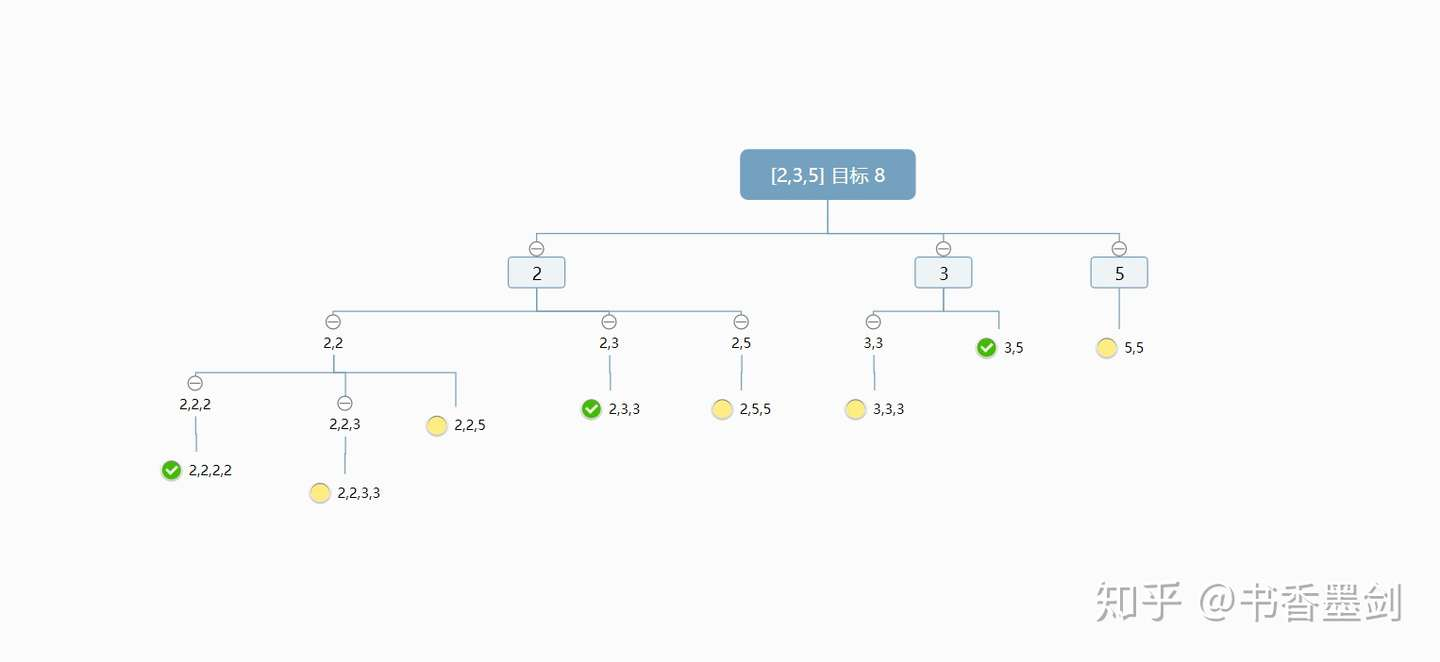【青铜三人行】每周一题@组合总和

组合总和

candidates 中的数字可以无限制重复被选取。

[
,
[2,2,3]
]

[
[2,2,2,2],
[2,3,3],
[3,5]
]

思路解法

/**
* @param {number[]} candidates
* @param {number} target
* @return {number[][]}
*/
function combinationSum(candidates, target) {
const result = [];
let tmpPath = [],
start = 0;

candidates = candidates.sort((a, b) => a - b);

function backtrack(tmpPath, target, start) {
if (target === 0) {
result.push(tmpPath);
return;
}

for (let index = start; index < candidates.length; index++) {
if (target < 0) break;

tmpPath.push(candidates[index]);
backtrack(tmpPath.slice(), target - candidates[index], index);
tmpPath.pop(); //回溯
}
}
backtrack(tmpPath, target, start);

return result;
}

function combinationSum(candidates, target) {
const sliceArr = candidates
.filter(item => item <= target)
.sort((a, b) => a - b),
finalArr = [];

function findCompose(target, offset, last) {
for (let i = offset; i < sliceArr.length; i++) {
const subTarget = target - sliceArr[i];
if (subTarget == 0) finalArr.push([...last, sliceArr[i]]);
if (subTarget > 0) findCompose(subTarget, i, [...last, sliceArr[i]]);
}
}
findCompose(target, 0, []);

return finalArr;
}

曾大师 Go 语言时间

// 深度搜索加减枝,具体过程如下
// 2 -> 22 -> 222 -> 2222 -> 223(合适) -> 23 -> 233 -> 26 -> 3 -> 33 -> 333 -> 36 -> 6 -> 66 ->7(合适)

var result [][]int
var currCandidate []int

func combinationSum(candidates []int, target int) [][]int {
sort.Ints(candidates)

result=make([][]int,0)
currCandidate=make([]int,0)

DFS(target,candidates)

return result
}

func DFS(target int,candidates []int) int {
if getSum(currCandidate) == target {
temCandidate := make([]int, len(currCandidate))
copy(temCandidate, currCandidate)
result = append(result, temCandidate)
return 0
} else if getSum(currCandidate) > target {
return -1
} else { //主要看这里用0代表相同，-1代表已经超过了当前target，1则表示还能继续加
for i := 0; i < len(candidates); i++ {
currCandidate = append(currCandidate, candidates[i])
temp := DFS(target, candidates[i:])
currCandidate = currCandidate[:len(currCandidate) - 1]
if temp <= 0 {
break
}
}
}
return 1
}

func getSum(nums []int) int {
sum := 0
for i := 0; i < len(nums); i++ {
sum += nums[i]
}
return sum
}

结语

OK，这样看下来，其实算法离我们也没有那么远。事实上如此，算法本身也是为了解决具体的问题而诞生的。而我们在练习的过程中，要理解到算法具体解决了什么问题，就可以在遇到类似的问题的时候迎刃而解啦~